## How to Choose Learning Tracks

Click to see a document showing all the problems learned in each of the 16 Learning Tracks. Click to see the problems in the tracks.

In the Rocket Math Online Game every student needs to be started in one of the operations or Learning Tracks. A student’s Learning Track can be changed at any time**, but one must be chosen to begin with.  Scroll down for “How to assign Learning Tracks.”

### Considerations, or what to choose when?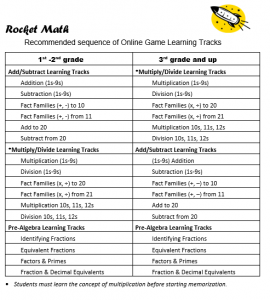Note: Compared to the Worksheet Program, the Online Game moves students through the Learning Tracks much faster–in a few weeks, rather than months.  Therefore you can send everyone of the same age through in the same order.

• Subtraction (1s – 9s)
• Fact Families (+, -) to 10.
• Fact Families (+, -) from 11.
• Subtract from 20.

Before going on any further, you should ensure that your students understand multiplication.  To know if they understand multiplication ask them to “figure out” the answer to a couple of multiplication facts such as 7 times 8 or 6 times 9.

If they cannot figure out the answer, then teach them the concept before letting them start memorizing multiplication facts.

If they have a process for determining the answer–they understand the operation and are ready to continue this sequence.

### Followed by Multiply/Divide Learning Tracks

• Multiplication (1s – 9s)
• Division (1s – 9s)
• Fact Families (x, ÷) to 20.
• Fact Families (x,÷) From 21.
• Multiplication 10s-11s-12s
• Division 10s-11s-12s

### Then followed by Pre-Algebra Learning Tracks

• Identifying Fractions
• Equivalent Fractions
• Factors & Primes
• Fraction & Decimal Equivalents.

### Grades 3 and up–Multiply/Divide Learning Tracks have priority.

We are assuming that the concept of multiplication has been taught first. (See above to check on readiness for multiplication facts.)

• Multiplication (1s – 9s)
• Division (1s – 9s)
• Fact Families (x, ÷) to 20.
• Fact Families (x,÷) From 21.
• Multiplication 10s-11s-12s
• Division 10s-11s-12s

### Then come back to Add/Subtract Learning Tracks

Once multiplication and division are mastered, 3rd grade and above can go back to pick up addition and subtraction.

• Subtraction (1s – 9s)
• Fact Families (+, -) to 10.
• Fact Families (+, -) from 11.

### Then the Pre-Algebra Learning Tracks.

• Identifying Fractions
• Equivalent Fractions
• Factors & Primes
• Fraction & Decimal Equivalents.

## We believe the Pre-Algebra Tracks are unique to Rocket Math.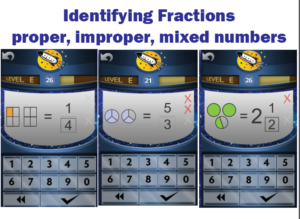Most valuable after the basics in grade 3, 4, 5 and up, in this order:

### (13) Identifying Fractions

• Learning to name fractions shown in a picture.  Students learn from examples of proper fractions, improper fractions (fractions equal to more than 1), and mixed numbers.

###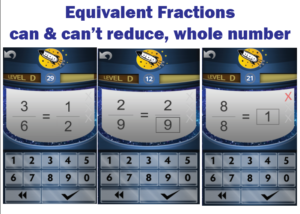(14) Equivalent Fractions

• Learning by memory the lowest terms of common fractions is very valuable.  Students also learn a lot of fractions that can’t be reduced to lower terms, such as 2/9 =2/9 or 1/4 = 1/4.  They learn to identify many fractions that are equal to one.

###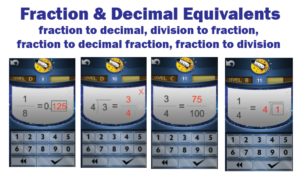(16) Fraction & Decimal Equivalents.

• Learning common fractions and their decimal equivalents, learning fractions and their fraction equivalents in 10ths or 100ths,  also learning to change a fraction into a division problem and the reverse.

**See “How to change Learning Tracks” in the FAQs and Directions document.

## How to assign Learning Tracks

### Auto-created Logins–and assignment of Learning Tracks.

You can have the system “auto-create” student logins (usernames and passcodes) and then assign them all to a Learning Track.  When you’re done you can just export the result. Watch this video to see how this works.

### D-I-Y created Student Logins (up to 30 at a time).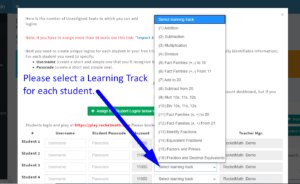If you are entering the Student Login with the D-I-Y Student Logins popup, you can use the pull down menu to select a learning track, for each student as illustrated. You can then assign a different learning track to each student.

### Entering more than 30 students using a CSV file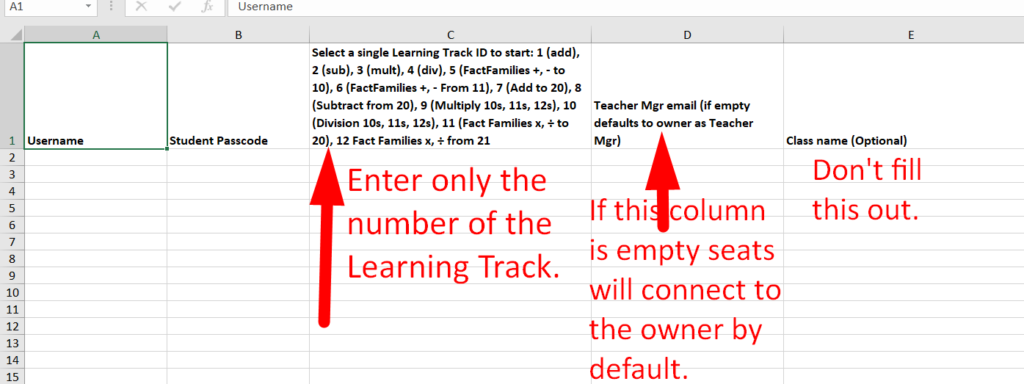If you are entering the Student Login using the csv method of entry you’ll be using the template we provide.  In column C do NOT enter the names of the learning tracks.  You must enter a number for each Learning Track in column C. The template gives you the numbers at the top of column C.  The learning tracks are numbered as follows.

2. Subtraction 1s through 9s
3. Multiplication 1s through 9s
4. Division 1s through 9s
5. Fact Families (+, -) to 10, ex.4+6, 6+4, 10-4, 10-6
6.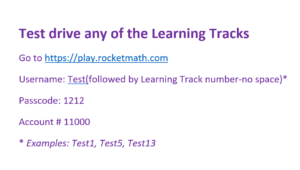Fact Families (+, -) from 11, ex. 5+6, 6+5, 11-6, 11-5.
7. Add to 20, example 13+4, 4+13,
8. Subtract from 20, example 15-3, 15-12,
9. Multiplication 10s-11s-12s,
10. Division 10s-11s-12s.
11. Fact Families (x, ÷) to 20, example 4×5, 5×4, 20÷4, 20÷5
12. Fact Families (x, ÷) from 21,example 3×7, 7×3, 21÷3, 21÷7
13. Identifying Fractions (proper, improper and mixed numbers)
14. Equivalent Fractions, example 3/6 = 1/2)
15. Factors & Primes, finding next pair of factors and knowing when done.
16. Fraction & Decimal Equivalents, e.g 1/8 = 0.125, 1/8 = 125/1000, 8 divided into 1 equals 1/8 and vice versa

This entry was posted in . Bookmark the permalink.